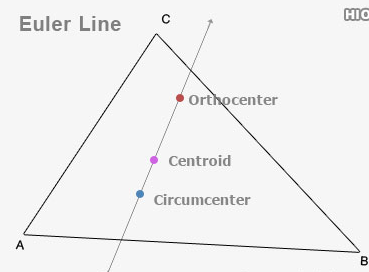# If orthocentre and circumcentre  of a  are respectively (1,1) and (3,2) , then the coordinates of its  centroid  are  Option 1) Option 2) Option 3) Option 4) None

D Divya Saini

Euler line -

In any non equilateral triangle the circumcentre (O), the centriod (G) and the orthocentre (H) are collinear and G divides OH in ratio 1:2

- whereinCr = Centroid; Orthocentre Circumcentre

Option 1)

This solution is correct

Option 2)

This solution is incorrect

Option 3)

This solution is incorrect

Option 4)

None

This solution is incorrect

Exams
Articles
Questions